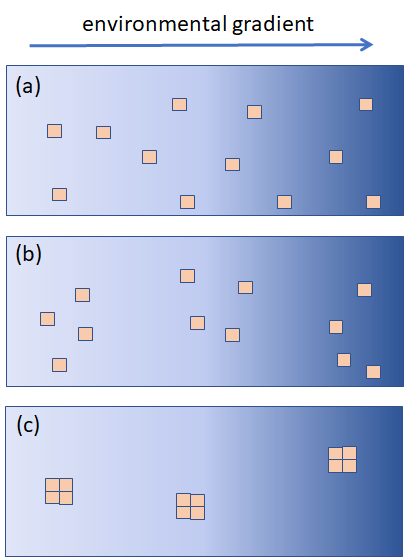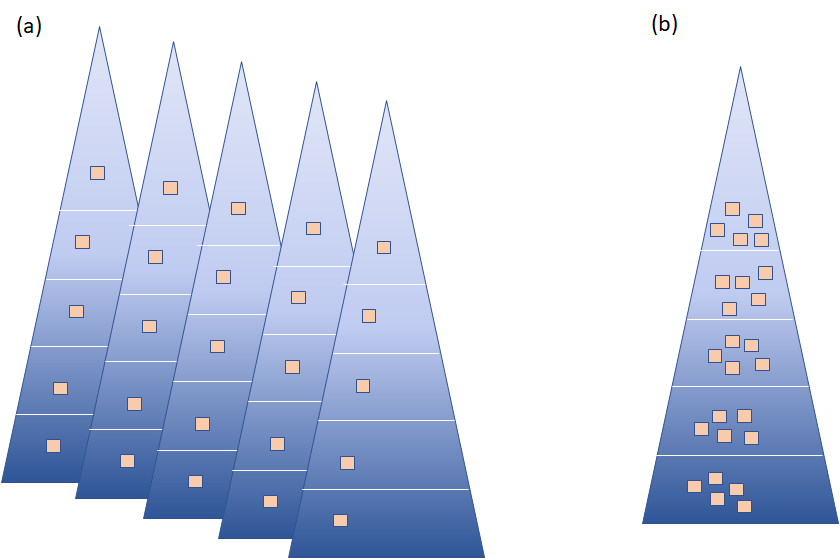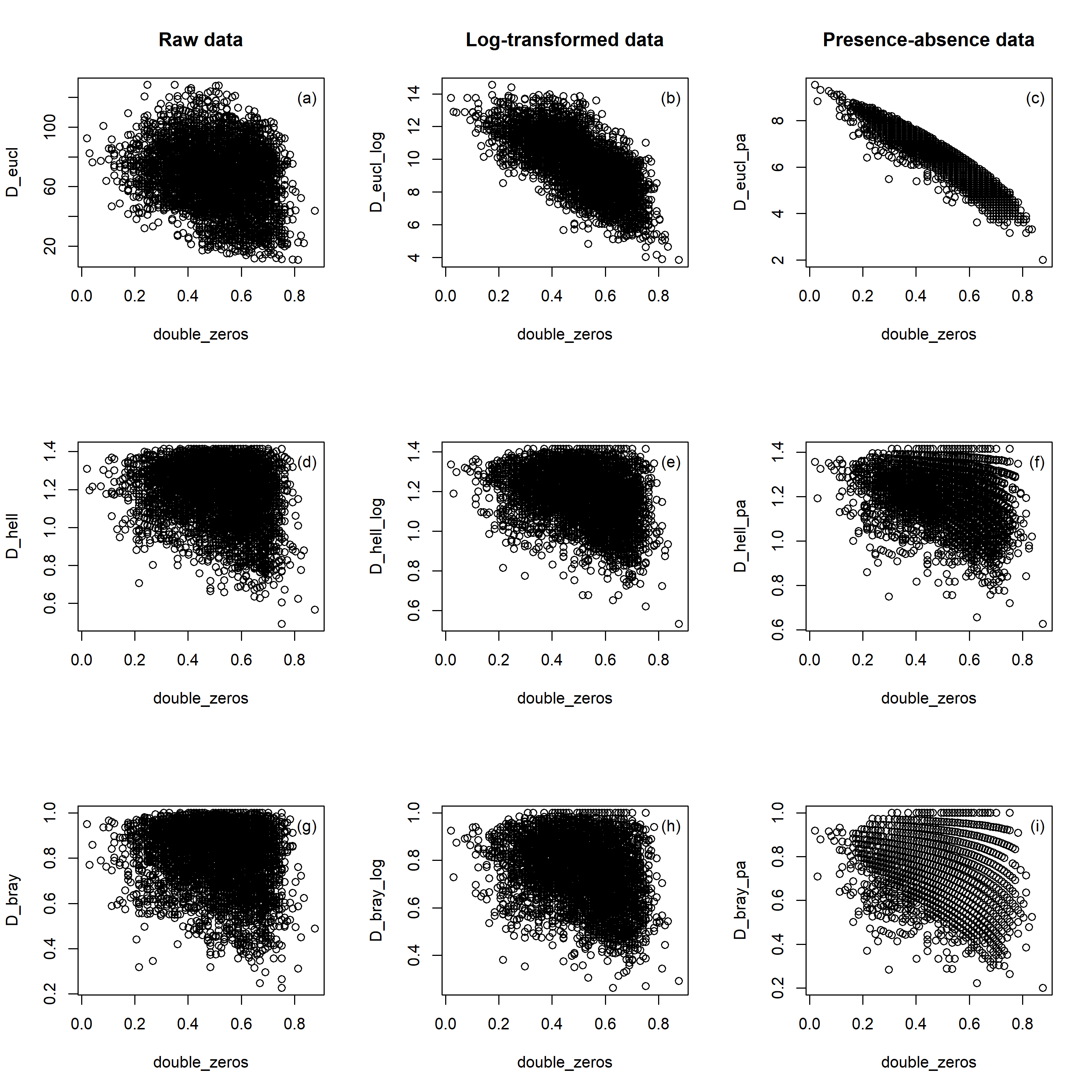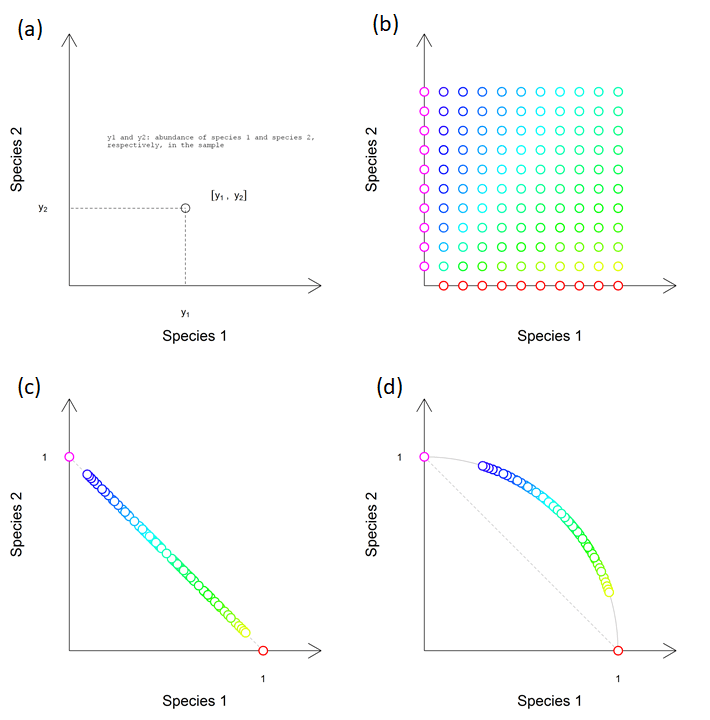# In observational ecological studies, pseudoreplications caused by spatial autocorrelation are more tricky than we may think

Simply put, pseudoreplications are replicates in the study that increase the sample size (n) but do not increase the information amount. A typical problem is if we treat pseudoreplications as independent samples, assuming that each contributes a new piece of information (and hence the full degree of freedom). Here, the problem is equivalent to the issue of spatial autocorrelation in the data: the sample close to the focal one is likely to be more similar than a random sample selected from the dataset, and is therefore somewhat a pseudoreplication. One of the consequences is an inflated Type I error rate in the significance test, with results more optimistic than would be warranted by data. Sometimes a way more optimistic – see below.

Why did I write this post? Because of my recent experience as a reader of scientific papers, a reviewer and also an editor. I encountered repeatedly situations when authors analyzed their data with clear pseudoreplications as if all samples are independent. I even saw a few papers published in nice journals that claim an effect of a specific environmental variable on species richness/composition, while the effect in reality was just a result of inflated Type I error rate. (How do I know that? The authors provided the original data used for their analysis, and I could show that even if I randomize their environmental variable while keeping its hierarchical structure, I have a high probability to obtain significant results with otherwise completely irrelevant effects). Last but not least, I write it also as a potential author of papers with flawed results because I am not being honest enough (with myself and others) that I have a pseudoreplication issue in my own data.

## Some background first

In experimental ecological studies, pseudoreplications are not that common, and if they appear, they usually result from a wrong sampling design and authors get eventually punished for them by reviewers. Less clear is the situation in observational ecological studies, based on field sampling. Look at the figure below for an example. Imagine that you want to study the change in diversity or species composition of some community (sampled within small square plots) along some environmental gradient (with intensity changing from left to right, bright to dark). Panel (a) shows a situation when individual samples are somewhat randomly distributed, in panel (b) they are somewhat clustered, and in panel (c) they are strongly clustered into three groups. We intuitively feel that the number of independent samples in panel (a) is close to 12, in panel (c) close to 3, and in panel (b) somewhere in between. In terms of pseudoreplications, while in panel (a) the samples are practically independent, in panel (c) the samples within the same group are almost complete pseudoreplications; they will likely have similar biological and environmental values since they are sampled in close vicinity of each other.Schema of three sampling designs along an environmental gradient. Samples (squares) are located either somewhat randomly or evenly (panel a), somewhat clustered (b) or highly clustered (c). The pseudoreplication issue and level of spatial autocorrelation tend to increase from (a) to (c).

When analyzing data with some level of pseudoreplication, or spatial autocorrelation, we need to consider the hierarchical structure of the data in the model or modify the test of significance accordingly. In the situation of randomly distributed samples (panel a), we may think it is not necessary, especially if samples are far enough (but what is far enough?). In the situation of strong clustering (panel c), we would perhaps directly include “block” into the model, effectively reducing our sample size to the number of blocks. But most situations are somewhat in the middle; there is some level of clustering, and we are left not knowing how big a problem this could be. Since doing a simple analysis (such as linear regression) is much easier than the analysis corrected for spatial autocorrelation (such as spatial autoregressive models), we have good reason to slightly close our eyes and ignore the problem.

## Numerical example with richness along elevation studied on one or several mountains

The example below illustrates the problem that pseudoreplications can cause if not accurately treated in the test of significance. Imagine that we study the pattern of richness (e.g. tree species) along elevation (see figure below). Each mountain (triangle) is separated into elevation zones (five in this example), and in each zone, we collect one or several samples and in each count the numbers of tree species. The figure below shows two scenarios, both generating datasets with 25 samples. In the first scenario (panel a) are five mountains, and each elevation zone in each mountain has only one sample. In the second scenario (panel b) is only one mountain, and each elevation zone in this mountain has five samples. Samples in the same elevation zone, but in a different mountain, may be considered as independent samples: they are far from each other, and their richness can be quite different (e.g. affected by other, regionally specific effects). In contrast, samples in the same elevation zone and the same mountain are clear pseudoreplications, since they are located close to each other and their richness is possibly rather similar (influenced by the same regional factors). For purpose of this example, let’s assume that different elevation zones on the same mountain are far from each other and are not spatially dependent (mountains are in reality not that sharp).Two scenarios of studying richness along elevation: (a) one sample per elevation zone, in five mountains, (b) five samples per elevation zone, in only one mountain. Both datasets contain 25 samples, but replicates at the same elevation zone in the same mountain are likely to be pseudoreplications.

Let’s extend this example, add one more scenario and some math. We add the third scenario (not on the figure above, since I was lazy to click in PowerPoint), which again has only one mountain, but each elevation zone has 100 samples, increasing the sample size to 500 samples. And now the math: we will analyze the correlation between richness and elevation while generating the richness values of individual samples randomly (i.e. creating a dataset where the null hypothesis, that richness and elevation are not dependent, is true, and our test should not reject it – or at least nor reject too often).

For scenario 1 (five mountains, one sample per each of the five elevation zones, n = 25), I assume that samples in the same elevation zone but on different mountain are independent, and I generated species richness of each sample as a random value from a uniform distribution between 0 and 100. For scenario 2 (one mountain, five samples per each of the five elevation zones, n = 25), I assume that samples in the same elevation zone and the same mountain will not be completely independent and will have rather similar richness values, since the richness (along to elevation) may be affected also by some locally specific factors (e.g. deforestation of the locality in the past). I first generated a richness value for each elevation zone (five random numbers from a uniform distribution between 0 and 100); each sample within the same elevation zone will get the richness of that zone plus randomly generated value (normal distribution, mean zero, sd = 10); in this way, the richness of samples within the same elevation zone slightly different (but not very much). For scenario 3 (one mountain, 100 samples per each of the five elevation zones, n = 500) I repeated the same as for scenario 2, but increased the number of samples within the same elevation zone to 100.

One example for each scenario is shown in the figure below (panel a, b and c for scenarios 1, 2 and 3, respectively). The x-axis shows hypothetical elevation (from 200 to 1000 m asl), and the y-axis shows the species richness of individual samples. I calculated the correlation between richness and elevation for each scenario and recorded whether the correlation t-test was significant at P < 0.05 or not. I repeated the simulation 100 times and counted how many significant results I get for each scenario (out of 100). Since we know that the null hypothesis is true (because we generated our data as such), and we decided that Type I error rate of 5% is sufficiently low to consider the result as sufficiently different (we consider P values lower than 0.05 as significant), we can expect that 5% of the tests for each scenario will be false positives (around 5 out of 100).

Panel (d) shows the number of results significant at P < 0.05, compared to the expectation (5%). While for scenario 1 the number fits the expectation (is around the proportion of 5%), scenario 2 has almost 50% of significant results, and scenario 3 almost 90%.

## Few notes at the end

The conclusion from the numerical example above could be: if you want to show that richness is related to elevation (even though it maybe does not), do not bother with climbing too many mountains, just try hard to make as many samples as you can on just one of them! But God bless you with convincing reviewers and readers that all your samples are independent replicates and they can trust your simple correlation results…

The more general suggestion would be: if your sampling design is like the panel (a) or (b) in the first schema above, check for autocorrelation in residuals of your model, and if it is there, be honest and correct for it, or at least report that it may possibly be a problem but you haven’t corrected for it (and why). If your design resembles the situation on panel (c), analyze your data directly as the block design (e.g. using linear mixed effect models with blocks as random effects). If you do not, while possibly having many replicates within each “block”, you are likely to hugely lie with your P-values. Highly significant but very small effect size (like low R2) will for sure cast doubts on your results.

# Euclidean distance is sensitive to double zero problem, while Hellinger is not: visualization

One obstacle to analysing community ecology data with linear ordination methods (principal component analysis, redundancy analysis) is their reliance on Euclidean distances to quantify compositional differences among communities (sites). Euclidean distance is known to be sensitive to double zero problem, i.e. species that are missing in the species composition of both compared communities. There exists a reasonable argument that for species composition data (occurrences of species in different communities), the absence of species in both compared communities does not really reveal meaningful information about their ecological resemblance. The species may be missing because the environment of each community is outside of the ecological niche of the species, but then both communities could be at the same end of the gradient (e.g. both too dry for species to occur, and hence environmentally quite similar) or each community on the other end of the gradient (one too dry, the other too wet, hence environmentally quite different). Distances that can be meaningfully applied for species composition data should preferentially ignore double zeros (be “asymmetric” – treat differently double presence and double absence). In the case of relatively homogeneous species composition data (with minimum double zeros) the Euclidean distance can be useful.

In the numerical example below, I will show the comparison of Euclidean and Hellinger distance in the context of the double zero problem. This comparison will show that Euclidean distance is really affected by the double zero problem, and the effect is strongest when the species composition data are transformed (by log or even presence-absence transformation). Hellinger distance, in contrast, is claimed as not sensitive to the double zero problem, and the illustration below clearly illustrates this feature. For comparison, I included also the third distance metric, Bray-Curtis, which is a quantitative version of Sørensen similarity and is known to be asymmetric (ignoring double zeros).

I took an example dataset about forest vegetation sampled in the Vltava river valley (Czech Republic), which is compositionally rather heterogeneous (and therefore contains a large number of double zeros). Dissimilarity (Euclidean, Hellinger and Bray-Curtis) is calculated among each pair of the 97 vegetation plots (communities). Abundances in these plots were either used raw (percentage cover of individual plant species estimated in the field), log-transformed as log (x+1), or transformed into presences and absences. The proportion of double zeros was for each pair of communities calculated as the number of species jointly missing in both compared communities, divided by the number of all species in the whole dataset (i.e. not only species present in the two communities, but all species present in all 97 vegetation plots).

I displayed the relationship of the proportion of double zeros (x-axis) against each dissimilarity metric (y-axis), with Euclidean, Hellinger and Bray-Curtis distance in the upper, middle and lower rows of the figure below, respectively, and applied on data either untransformed (first column), log-transformed (middle column) or transformed into presences-absences (right column). I have not calculated any statistic to quantify the relationship between distances and proportions of double zeros, and haven’t tested it, since individual data points represent pairwise comparisons among pairs of communities in the dataset, and some of them share the same community (and are therefore not independent).

The results show that Euclidean distance is related to the proportion of double zeros in the community, and this relationship is very strong if the original species composition data were transformed into presences-absences (but is also obvious for log-transformed data, less for untransformed). Hellinger distance, in contrast, is not directly related to the proportion of double zeros. In the case of Hellinger distance, the pattern appears a bit triangular: if the proportion of double zeros is low (i.e. the communities share most of the species), calculated dissimilarity intuitively cannot be too low; on the other side, if the double zero proportion is high, the dissimilarity can be both low or high (depending on which species are being shared among communities). Bray-Curtis distance behaves very similarly to the Hellinger distance; in fact, the Hellinger and Bray-Curtis distances are rather correlated, and this correlation is very strong when calculated from presence-absence data (pattern not shown here).The relationship of the dissimilarity between the pair of communities (y-axis, either Euclidean, D_eucl, or Hellinger, D_hell, or Bray-Curtis, D_bray) and the proportion of double zeros in the compared pair of communities (x-axis, double_zero).

In this context, I also focused on Hellinger standardization (or transformation, call it as you wish). The reason is that Hellinger distance between pair of communities can be calculated by taking the raw species composition data (with original or transformed abundances, e.g. by log or sqrt transformation), and applying the Hellinger standardization on it (which involves relativization of abundances within the community by dividing abundance of each species by the sum of abundances in the community, and then by square-rooting the relativized value). If we apply Euclidean distance on such Hellinger standardized raw data, we will get Hellinger distance.

I visualized the process of standardization in the figure below. Panel (a) shows the concept of two-dimensional species space, where each dimension is defined by an abundance of one species (species 1 on the x-axis and species 2 on the y-axis). Communities (circles) are located in this space according to the abundance of the two species. Panel (b) shows the distribution of 120 samples (communities) in the two-species space. Absolute values of the abundances are not relevant, important is that the units on the x- and y-axis are the same; e.g., in the yellow sample which is at the second row from the bottom the most at the right, the species abundances could be [100, 20], [10, 2], or [1, 0.2]. Panel (c) shows the result of the first step in Hellinger standardization, which is the calculation of “species profiles” (species abundances is standardized by row sums, i.e. the sum of species abundances in its community). Samples standardized to species profiles are located on the hypotenuse of the right-angled triangle with corner coordinates [0,0], [0,1] and [1,0]. Panel (d) shows the position of samples after the second step of Hellinger standardization, square rooting of species profiles; all samples are located at the perimeter of the circle, which has a radius of 1.

What is interesting on Hellinger-standardized abundance values is that communities with the same colour in the original two-species space have the same abundance after standardization.Visualization of Hellinger standardization. (a) Position of the community sample in the two-dimensional space defined by species 1 and species 2 is defined by the abundances of these species in the community. (b) Distribution of 120 communities with different abundances of species 1 and species 2. (c) Position of communities after standardization to “species profiles” (first step of Hellinger standardization) and (d) square-rooting (the second step of the standardization). The animation of the whole process can be seen here: https://vimeo.com/689244325/339fd4c86e